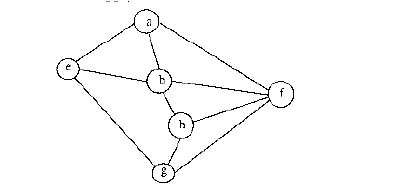Related Articles

# GATE | GATE-CS-2003 | Question 21

• Last Updated : 15 Nov, 2018

Consider the following graph,Among the following sequences:

```(I) a b e g h f
(II) a b f e h g
(III) a b f h g e
(IV) a f g h b e  ```

Which are depth first traversals of the above graph?
(A) I, II and IV only
(B) I and IV only
(C) II, III and IV only
(D) I, III and IV only

Explanation: DFS of a graph

```1) Visits a node.
2) Do following for every unvisited adjacent.
a) Completely explores all vertices through current
adjacent using recursive call to DFS.
```

There can be any DFS possible as we may pick different vertices as starting points and we may pick adjacents in different orders.

(i) a b e g h f [Visit a, explore all adjacents through b, and so on..]. In this b’s adjacent e is picked first
(iii) a b f h g e [Visit a, explore all adjacents through b, and so on..]. In this b’s adjacent f is picked first
(iv) a f g h b e [Visit a, explore all adjacents through f, and so on..]. In this f’s adjacent g is picked first

(ii) a b f e h g can not be an answer as e is visited after f [e is not an adjacent of f and all adjcents of f are not explored yet]

Quiz of this Question

Attention reader! Don’t stop learning now.  Practice GATE exam well before the actual exam with the subject-wise and overall quizzes available in GATE Test Series Course.

Learn all GATE CS concepts with Free Live Classes on our youtube channel.

My Personal Notes arrow_drop_up